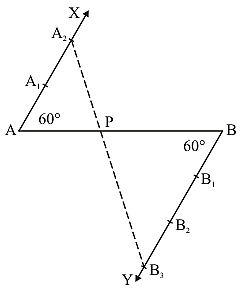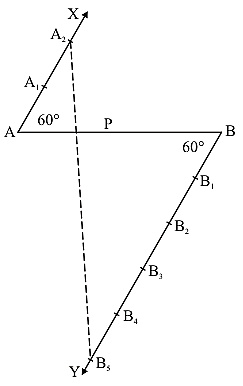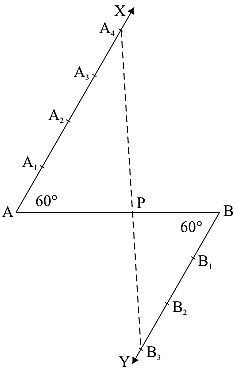# RD Sharma Class 10 Ex 11.1 Solutions Chapter 11 Constructions

In this chapter, we provide RD Sharma Class 10 Ex 11.1 Solutions Chapter 11 Constructions for English medium students, Which will very helpful for every student in their exams. Students can download the latest RD Sharma Class 10 Ex 11.1 Solutions Chapter 11 Constructions pdf, Now you will get step by step solution to each question.

# Chapter 11: Constructions​ Exercise – 11.1

### Question: 1

Determine a point which divides a line segment of length 12 cm internally in the ratio of 2: 3. Also, justify your construction.

### Solution:

Steps of Construction:1. Draw a line segment AB of 12 cm

2. Through the points A and B draw two parallel line on the opposite side of AB

3. Cut 2 equal parts on AX and 3 equal parts on BY such that AX1 = X1X2 and BX= Y1Y2 = Y2Y3.

4. Join X2Y3 which intersects AB at P

∴  AP/PB = 2/3.

Justification:

In ΔAX2P and ΔBY3P, we have

∠APX2 = ∠BPY3 {Because they are vertically opposite angle}

∠X2AP = ∠Y3BP {Because they are alternate interior angles}

ΔAX2P ΔBY3P {Because AA similarity}

∴  AP/BP = AX2/BY= 2/3 {Because of C.P.C.T}

### ​Question: 2

Divide a line segment of length 9 cm internally in the ratio 4 : 3. Also, give justification for the construction.

### Solution:

Steps of construction:1. Draw a line segment AB of 9 cm

2. Through the points, A and B, draw two parallel lines AX and BY on the opposite side of AB

3. Cut 4 equal parts on AX and 3 equal parts on BY such that: AX1 = X1X2 = X2X= X3X4 and BY1 = Y1Y2 = Y2Y3

4. Join X4Y3 which intersects AB at P

∴  AP/PB = 4/3

Justification:

In ΔAPX4 and ΔBPY3, we have

∠APX4 = ∠BPY3 {Because they are vertically opposite angles}

∠PAX4 = ∠PBY3 {Because they are alternate interior angle}

ΔAPX4 ΔBPY3 {Because AA similarity}

∴  PAPB = AX4/BY3 = 4/3 {Because of C.P.C.T}

### ​Question: 3

Divide a line segment of length 14 cm internally in the ratio 2 : 5. Also, give justification for the construction.

### Solution:

Steps of construction:1. Draw a line segment AB of 14 cm

2. Through the points A and B, draw two parallel lines AX and BY on the opposite side of AB

3. Starting from A, Cut 2 equal parts on AX and starting from B, cut 5 equal parts on BY such that: AX1 = X1X2 and BY1 = Y1Y2 = Y2Y3 = Y3Y4 = Y4Y5

4. Join X2Y5 which intersects AB at P

∴  AP/PB = 2/5

Justification:

In ΔAPX2 and ΔBPY5, we have

∠APX2 = ∠BPY5 {Because they are vertically opposite angles}

∠PAX2 = ∠PBY5 {Because they are alternate interior angles}

Then, ΔAPX2 ΔBPY5 {Because AA similarity}

∴  AP/PB = AX2/BY5 = 2/5 {Because of C.P.C.T}

All Chapter RD Sharma Solutions For Class10 Maths

I think you got complete solutions for this chapter. If You have any queries regarding this chapter, please comment on the below section our subject teacher will answer you. We tried our best to give complete solutions so you got good